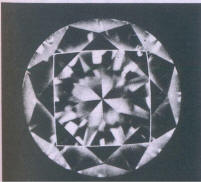## Table comparison method

In observing the lines of the table-edges - they are formed by the table-edges and in their elongation by the edges of the table facets - it can be seen that these, according to the size of the table, may be convex, straight or concave (Fig 211 and 212). The smaller the table the more convex are the table-edge lines, that is, curved towards the centre of the table, and then correspond to a table size of between 54 and 59 % of the girdle diameter (Fig 213 and 213a ).Fig 213 Convex table edge: table size about 56% Fig 213a Convex table edge: table size about 58%